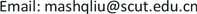Hindmarsh-Rose模型，李雅普诺夫函数，全局渐近稳定, Hindmarsh-Rose Model Lyapunov Function Global Asymptotic Stability1. 引言

2. 模型描述2.1. 单个HR模型

HR模型是Hindmarsh和Rose根据电压钳实验获得的关于蜗牛神经细胞数据而提出的，它能模拟软体动物神经元重复的峰放电和不规则的簇放电行为，是一类可兴奋的神经元模型。其简化的神经元模型有利于模拟真实神经网络，单个HR模型描述如下：

{ d x 1 d t = x 2 + f ( x 1 ) − x 3 + I , d x 2 d t = g ( x 1 ) − x 2 , d x 3 d t = μ [ s ( x 1 − χ ) − x 3 ] , (1)

2.2. N个神经元电耦合的动力系统

{ d x i 1 d t = f 1 ( X i ) + ∑ j = 1 N k i j g i j x j 1 , d x i 2 d t = f 2 ( X i ) , d x i 3 d t = f 3 ( X i ) , i = 1 , 2 , 3 , ⋯ , N, (2)

d X i d t = F ( X i ) + k ∑ j = 1 N g i j T X j , i = 1 , 2 , 3 , ⋯ , N , (3)

X 1 ( t ) = X 2 ( t ) = ⋯ = X N ( t ) = s ( t ) , t → + ∞ , (4)

d γ d t = ( D X F ( s ( t ) ) + k λ m T ) γ , m = 2 , 3 , ⋯ , N , (5)

3. 三种连接方式分析

3.1. 链式连接

d V d t = γ T ⋅ ( [ D F ( s ( t ) ) ] T ⋅ A + A ⋅ D F ( s ( t ) ) + 2 k λ m A T ) ⋅ γ = γ T ⋅ [ f ′ ( x 1 ) + k λ m g ′ ( x 1 ) + 1 2 0 g ′ ( x 1 ) + 1 2 − 1 0 0 0 − 1 s ] ⋅ γ ≤ ( H + k λ m ) | γ 1 | 2 + ( L + 1 ) | γ 1 γ 2 | − | γ 2 | 2 − 1 s | γ 3 | 2 ≤ ( | γ 1 | , | γ 2 | , | γ 3 | ) ⋅ [ H + k λ m L + 1 2 0 L + 1 2 − 1 0 0 0 − 1 s ] ⋅ ( | γ 1 | , | γ 2 | , | γ 3 | ) T

3.2. 环状连接

3.3. 全局连接

4. 数值模拟

e ( t ) = | 1 N ∑ i = 1 N x i 1 ( t ) 2 − ( 1 N ∑ i = 1 N x i 1 ( t ) ) 2 | ,

e ( t ) 表示 t 时刻的同步差，它反映了耦合系统的同步状态。规定当耦合强度 k > k 0 时，如果 lim t → + ∞ e ( t ) = 0 ，那么称系统(3)达到了完全同步，此时我们把满足 k > k 0 条件的 k 0 的最小值称为耦合系统实际的同步阈值。

Data of the number of neuron and synchronization thresholds for three types of regular coupled neural network

4193.31.5656.620.4728.310.235
5296.42.4281.940.6522.650.179
6422.63.49113.250.9118.870.152
7571.74.68150.391.2116.170.131
8743.86.00193.321.5414.150.116
9938.97.58242.031.9112.580.101
101156.99.30296.492.3611.320.093
111397.911.1356.702.8410.290.082
121661.813.2422.653.369.4370.076

5. 本文结论